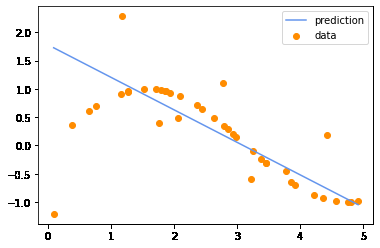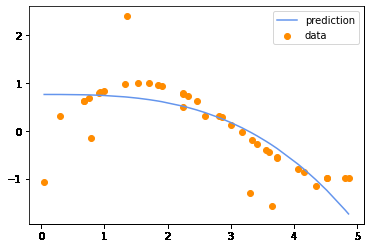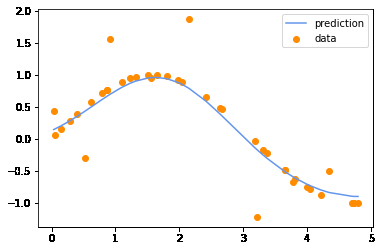# Support Vector Regression (SVR) using Linear and Non-Linear Kernels in Scikit Learn

Support vector regression (SVR) is a type of support vector machine (SVM) that is used for regression tasks. It tries to find a function that best predicts the continuous output value for a given input value.

SVR can use both linear and non-linear kernels. A linear kernel is a simple dot product between two input vectors, while a non-linear kernel is a more complex function that can capture more intricate patterns in the data. The choice of kernel depends on the data’s characteristics and the task’s complexity.

In scikit-learn package for Python, you can use the SVR’ class to perform SVR with a linear or non-linear kernel’. To specify the kernel, you can set the kernel parameter to ‘linear’ or ‘RBF’ (radial basis function).

### Concepts related to the Support vector regression (SVR):

There are several concepts related to support vector regression (SVR) that you may want to understand in order to use it effectively. Here are a few of the most important ones:

• Support vector machines (SVMs): SVR is a type of support vector machine (SVM), a supervised learning algorithm that can be used for classification or regression tasks. SVMs try to find the hyperplane in a high-dimensional space that maximally separates different classes or output values.
• Kernels: SVR can use different types of kernels, which are functions that determine the similarity between input vectors. A linear kernel is a simple dot product between two input vectors, while a non-linear kernel is a more complex function that can capture more intricate patterns in the data. The choice of kernel depends on the data’s characteristics and the task’s complexity.
• Hyperparameters: SVR has several hyperparameters that you can adjust to control the behavior of the model. For example, the ‘C’ parameter controls the trade-off between the insensitive loss and the sensitive loss. A larger value of ‘C’ means that the model will try to minimize the insensitive loss more, while a smaller value of C means that the model will be more lenient in allowing larger errors.
• Model evaluation: Like any machine learning model, it’s important to evaluate the performance of an SVR model. One common way to do this is to split the data into a training set and a test set, and use the training set to fit the model and the test set to evaluate it. You can then use metrics like mean squared error (MSE) or mean absolute error (MAE) to measure the error between the predicted and true output values.

### Fitting an SVR Model on the Sine Curve data using Linear Kernel

First, we will try to achieve some baseline results using the linear kernel on a non-linear dataset and we will try to observe up to what extent it can be fitted by the model.

## Python3

 `import` `numpy as np ` `import` `matplotlib.pyplot as plt ` `from` `sklearn.svm ``import` `SVR ` ` `  `# generate synthetic data ` `X ``=` `np.sort(``5` `*` `np.random.rand(``40``, ``1``), ` `            ``axis``=``0``) ` `y ``=` `np.sin(X).ravel() ` ` `  `# add some noise to the data ` `y[::``5``] ``+``=` `3` `*` `(``0.5` `-` `np.random.rand(``8``)) ` ` `  `# create an SVR model with a linear kernel ` `svr ``=` `SVR(kernel``=``'linear'``) ` ` `  `# train the model on the data ` `svr.fit(X, y) ` ` `  `# make predictions on the data ` `y_pred ``=` `svr.predict(X) ` ` `  `# plot the predicted values against the true values ` `plt.scatter(X, y, color``=``'darkorange'``, ` `            ``label``=``'data'``) ` `plt.plot(X, y_pred, color``=``'cornflowerblue'``, ` `         ``label``=``'prediction'``) ` `plt.legend() ` `plt.show() `

Output:Model fitted using Linear kernel

### Fitting an SVR Model on the Sine Curve data using Polynomial Kernel

Now we will fit a Support vector Regression model using a polynomial kernel. This will be hopefully a little better than the SVR model with a linear kernel.

## Python3

 `import` `numpy as np ` `import` `matplotlib.pyplot as plt ` `from` `sklearn.svm ``import` `SVR ` ` `  `# generate synthetic data ` `X ``=` `np.sort(``5` `*` `np.random.rand(``40``, ``1``), axis``=``0``) ` `y ``=` `np.sin(X).ravel() ` ` `  `# add some noise to the data ` `y[::``5``] ``+``=` `3` `*` `(``0.5` `-` `np.random.rand(``8``)) ` ` `  `# create an SVR model with a linear kernel ` `svr ``=` `SVR(kernel``=``'poly'``) ` ` `  `# train the model on the data ` `svr.fit(X, y) ` ` `  `# make predictions on the data ` `y_pred ``=` `svr.predict(X) ` ` `  `# plot the predicted values against the true values ` `plt.scatter(X, y, color``=``'darkorange'``, ` `            ``label``=``'data'``) ` `plt.plot(X, y_pred, color``=``'cornflowerblue'``, ` `         ``label``=``'prediction'``) ` `plt.legend() ` `plt.show() `

Output:Model fitted using a polynomial kernel

### Fitting an SVR Model on the Sine Curve data using RBF Kernel

Now we will fit a Support vector Regression model using an RBF(Radial Basis Function) kernel. This will help us to achieve probably the best results as the RBF kernel is one of the best kernels which helps us to introduce non-linearity in our model.

## Python3

 `import` `numpy as np ` `import` `matplotlib.pyplot as plt ` `from` `sklearn.svm ``import` `SVR ` ` `  `# generate synthetic data ` `X ``=` `np.sort(``5` `*` `np.random.rand(``40``, ``1``), ` `            ``axis``=``0``) ` `y ``=` `np.sin(X).ravel() ` ` `  `# add some noise to the data ` `y[::``5``] ``+``=` `3` `*` `(``0.5` `-` `np.random.rand(``8``)) ` ` `  `# create an SVR model with a linear kernel ` `svr ``=` `SVR(kernel``=``'rbf'``) ` ` `  `# train the model on the data ` `svr.fit(X, y) ` ` `  `# make predictions on the data ` `y_pred ``=` `svr.predict(X) ` ` `  `# plot the predicted values against the true values ` `plt.scatter(X, y, color``=``'darkorange'``, ` `            ``label``=``'data'``) ` `plt.plot(X, y_pred, color``=``'cornflowerblue'``, ` `         ``label``=``'prediction'``) ` `plt.legend() ` `plt.show() `

Output:Model fitted using RBF kernel

Whether you're preparing for your first job interview or aiming to upskill in this ever-evolving tech landscape, GeeksforGeeks Courses are your key to success. We provide top-quality content at affordable prices, all geared towards accelerating your growth in a time-bound manner. Join the millions we've already empowered, and we're here to do the same for you. Don't miss out - check it out now!

Previous
Next